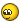We use cookies to ensure that we give you the best experience on our website. If you continue to use this site we will assume that you are happy with it.

# orbit camera collision / limits (feature request?)

Home Forums Programming orbit camera collision / limits (feature request?)

Viewing 9 posts - 1 through 9 (of 9 total)
• Author
Posts
• #35565

not sure if this feature exists, but we would like to keep the orbit camera from moving inside of objects.

so it would be a helpful feature to give it a collision material, similar to the first person camera.

an alternative would be using the Min / Max Angles, but since they are not visible in the viewport, it is hard to set them up correctly. a viewport gizmo/overlay/handles would be appreciated here.

#35576

made a short script, that shows the limits:

it adds a button to the UI: currently it is creating a mesh, with the near and far limits & angle limits.
downside: you have to undo / delete it & click the button again, every time you change a limit value.
upside: you dont have to export to find out

maybe this can be fleshed out & integrated into v3d natively

``````
# Author: Thomas Pahler @ UP Designstudio

import bpy, bmesh, mathutils, math

def draw():
camera = bpy.context.active_object

obj = bpy.context.scene.objects.get("debugCube")
if not obj:
mesh = bpy.data.meshes.new("debugCube")
obj = bpy.data.objects.new(mesh.name, mesh)
scene = bpy.context.scene
else:
mesh = obj.data

verts = []
edges = []
faces = []

# get camera limits
tgt = camera.data.v3d.orbit_target
pos = camera.location
camDirection = pos-tgt
near = camDirection.normalized()*camera.data.v3d.orbit_min_distance+tgt
far  = camDirection.normalized()*camera.data.v3d.orbit_max_distance+tgt

# add cross at target position
def drawCross (position):
verts.append(position-mathutils.Vector((0,1,0)))
verts.append(position+mathutils.Vector((0,1,0)))
verts.append(position-mathutils.Vector((1,0,0)))
verts.append(position+mathutils.Vector((1,0,0)))
verts.append(position-mathutils.Vector((0,0,0)))
verts.append(position+mathutils.Vector((0,0,1)))
drawCross(tgt)

#add line from near to far, in camera direction
verts.append(near)
verts.append(far)

count = 32

#add circle for horizontal limits at near limit distance
angle = camera.data.v3d.orbit_min_azimuth_angle
endAngle = camera.data.v3d.orbit_max_azimuth_angle
sweepAngle = endAngle-angle

for i in range(count):
angle += sweepAngle/count
verts.append(mathutils.Vector((math.sin(angle),-math.cos(angle),0)) *camera.data.v3d.orbit_min_distance + tgt)

#add circle for vertical limits at far limit distance
angle = camera.data.v3d.orbit_min_polar_angle
endAngle = camera.data.v3d.orbit_max_polar_angle
sweepAngle = endAngle-angle

for i in range(count):
angle += sweepAngle/count
verts.append(mathutils.Vector((0,-math.sin(angle),math.cos(angle))) *camera.data.v3d.orbit_max_distance + tgt)

# make edges
for i in range(int(len(verts)/2)):
edges.append([i*2,i*2+1])

mesh.from_pydata(verts, edges, faces)

#def emptyAtPosition (name, position, direction):
#    obj = bpy.context.scene.objects.get(name)
#    o = bpy.data.objects.new( "empty", None )

class DrawCameraLimits(bpy.types.Operator):
bl_idname = "v3d.draw_camera_limits"
bl_label = "Draw Limits"
bl_options = {"REGISTER", "UNDO"}

def execute(self, context):
draw()
return {'FINISHED'}

self.layout.operator(DrawCameraLimits.bl_idname)

bpy.utils.register_class(DrawCameraLimits)

#bpy.ops.v3d.draw_camera_limits()``````
#36311

we made an extension script, which draws the limits as a mesh.
it is very hacky as of now, but helps iterate faster on the limits.

a nicer implementation as overlay / tool / handle would be nice, but not sure how this is possible in blender 2.8+.

``````import bpy, bmesh, mathutils, math

def draw():
camera = bpy.context.active_object

obj = bpy.context.scene.objects.get("debugCube")
if not obj:
mesh = bpy.data.meshes.new("debugCube")
obj = bpy.data.objects.new(mesh.name, mesh)
scene = bpy.context.scene
else:
mesh = obj.data

verts = []
edges = []
faces = []

# get camera limits
tgt = camera.data.v3d.orbit_target
pos = camera.location
camDirection = pos-tgt
near = camDirection.normalized()*camera.data.v3d.orbit_min_distance+tgt
far  = camDirection.normalized()*camera.data.v3d.orbit_max_distance+tgt

# add cross at target position
def drawCross (position):
verts.append(position-mathutils.Vector((0,1,0)))
verts.append(position+mathutils.Vector((0,1,0)))
verts.append(position-mathutils.Vector((1,0,0)))
verts.append(position+mathutils.Vector((1,0,0)))
verts.append(position-mathutils.Vector((0,0,0)))
verts.append(position+mathutils.Vector((0,0,1)))
drawCross(tgt)

#add line from near to far, in camera direction
verts.append(near)
verts.append(far)

count = 32

#add circle for horizontal limits at near limit distance
angle = camera.data.v3d.orbit_min_azimuth_angle
endAngle = camera.data.v3d.orbit_max_azimuth_angle
sweepAngle = endAngle-angle

for i in range(count):
angle += sweepAngle/count
verts.append(mathutils.Vector((math.sin(angle),-math.cos(angle),0)) *camera.data.v3d.orbit_min_distance + tgt)

#add circle for vertical limits at far limit distance
angle = camera.data.v3d.orbit_min_polar_angle
endAngle = camera.data.v3d.orbit_max_polar_angle
sweepAngle = endAngle-angle

for i in range(count):
angle += sweepAngle/count
verts.append(mathutils.Vector((0,-math.sin(angle),math.cos(angle))) *camera.data.v3d.orbit_max_distance + tgt)

for i in range(int(len(verts)/2)):
edges.append([i*2,i*2+1])

mesh.from_pydata(verts, edges, faces)

#def emptyAtPosition (name, position, direction):
#    obj = bpy.context.scene.objects.get(name)
#    o = bpy.data.objects.new( "empty", None )

class DrawCameraLimits(bpy.types.Operator):
"""draw the camera limits as a 3d object"""
bl_idname = "object.draw_limits"
bl_label = "draw limits"
bl_options = {'REGISTER', 'UNDO'}

def execute(self, context):
draw()
return {'FINISHED'}

layout = self.layout
print("test")
layout.separator()
layout.operator(DrawCameraLimits.bl_idname)

classes=[ DrawCameraLimits ]

def register():
for c in classes:
bpy.utils.register_class(c)

def unregister():
for c in classes:
bpy.utils.unregister_class(c)

if __name__ == "__main__":
register()``````
###### Attachments:
You must be logged in to view attached files.
#36353

awesome! thanks for sharing this!Chief 3D Verger | LinkedIn | Twitter

#40372

since we use these limits quite a lot, i had a deeper look at it, and updated it to use gizmos.
they are not perfect (some range visual instead of two circles would be nicer), but they help a lot speeding up the worflow to set up and change limits.

we packed it as an installable plugin, so others can use it easily: download the .py and install via the blender preferences.

feel free to integrate this one in a future verge3d release, if wanted.

###### Attachments:
You must be logged in to view attached files.
#40374

second try to add the package, maybe a zip works?

###### Attachments:
You must be logged in to view attached files.
#40413

that will be super helpful, until now what ive been doing is locking camera to view locking camera on target then setting distance constraints + angle which gives you a locked in range that you can fly around and test out and adjust but seeing will be nice

#41051

Hi
Thomasup

that’s really a cool suggestion. This is something missing from verge as of now but will be cool addition for speeding up the work.

It will bypass adjusting parameters and checking them by publishing and again readjusting & then finalize.

#43347

second try to add the package, maybe a zip works?

Thanks for sharing Thomas. A short video clip showing and telling how to use this add-on would be helpful.

Again, thanks for sharing.

Viewing 9 posts - 1 through 9 (of 9 total)
• You must be logged in to reply to this topic.